>汽车>正文

# 念念不忘的IF函数和他的兄弟们全齐了

IF函数：判断条件是否成立，然后返回对应的结果；

AND函数：“和”的关系，判断两个参数是否同时成立，成立返回TURE,不成立返回FALSE;

OR函数：“或”的关系，判断两个参数只要有一个成立即返回TRUE，否则返回FALSE；

NOT函数：“非”的关系。

01

IF函数函数判断称谓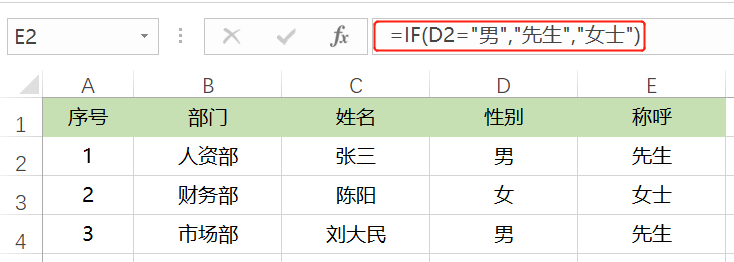02

IF函数的多层嵌套判断等级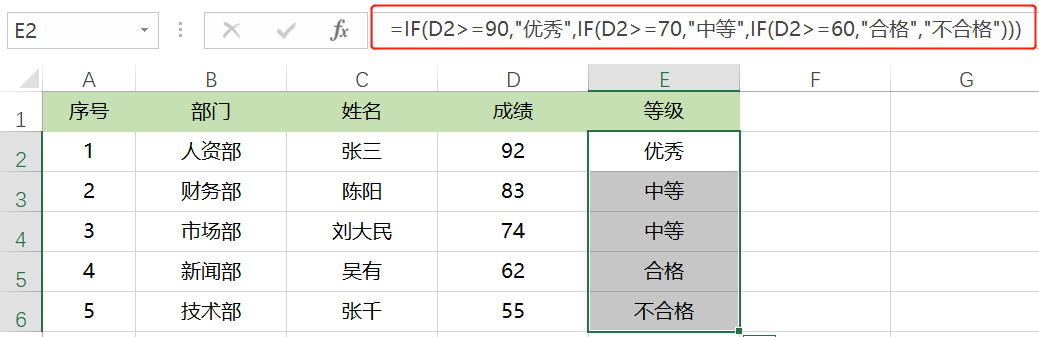=IF(D2>=90,"优秀",IF(D2>=70,"中等",IF(D2>=60,"合格","不合格")))，然后确定向下填充。

03

IF+AND函数配合判断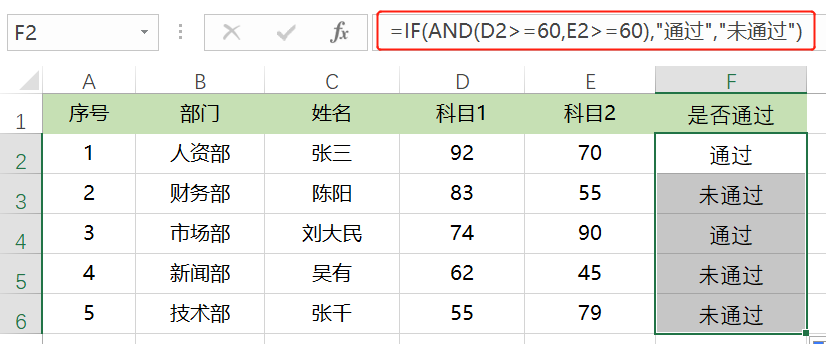=IF(AND(D2>=60,E2>=60),"通过","未通过")，然后确定向下填充。

04

IF+OR函数判断退休年龄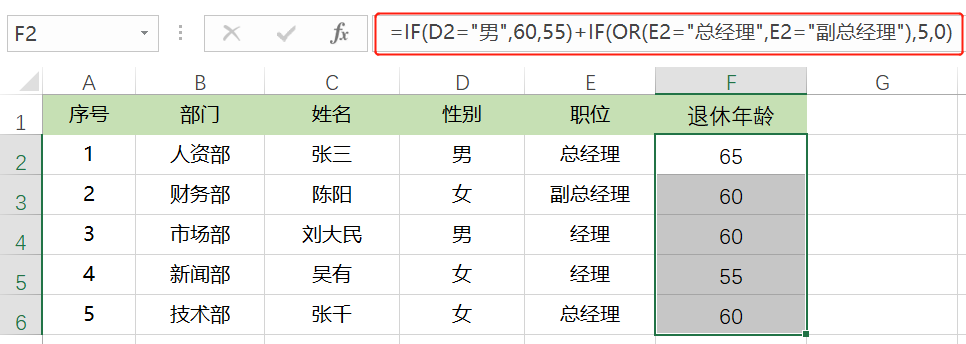=IF(D2="男",60,55)+IF(OR(E2="总经理",E2="副总经理"),5,0)，确定向下填充。

05

IF+NOT函数配合判断称谓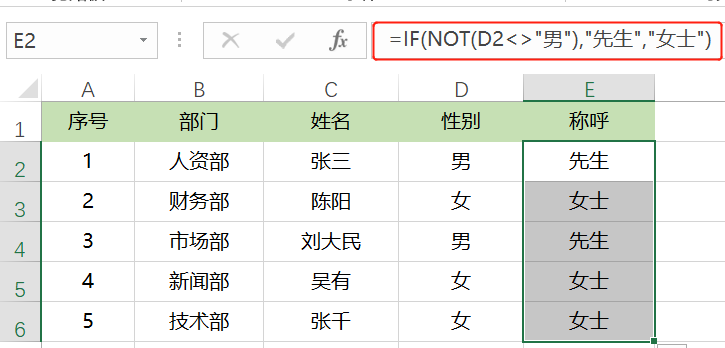=IF(NOT(D2<>"男"),"先生","女士")，确定向下填充。

06

IFERROR函数

IFERROR函数是屏蔽错误值的一个函数。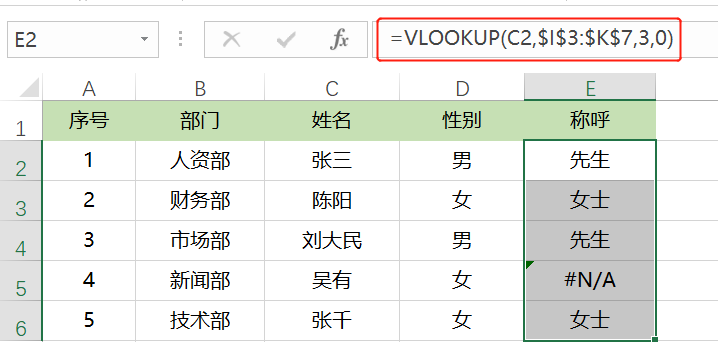=IFERROR(VLOOKUP(C2,\$I\$3:\$K\$7,3,0),"")，确定后向下填充。